The two basic lines of inquiry in statistical inference have long been: (i) to determine fundamental statistical (i.e., information-theoretic) limits; and (ii) to find efficient algorithms achieving these limits. However, for many structured inference problems, it is not clear if statistical optimality is compatible with efficient computation. Statistically optimal estimators often entail an infeasible exhaustive search. Conversely, for many settings the computationally efficient algorithms we know are statistically suboptimal, requiring higher signal strength or more data than is information-theoretically necessary. This phenomenon is both fascinating and unsettling. It suggests that the information-theoretic limit on the signal-to-noise ratio (or the amount of data) for these problems, as studied since the beginning of mathematical statistics, is not the practically relevant benchmark for modem high-dimensional settings. Instead, the practically relevant benchmark is the fundamental statistical limit for computationally efficient algorithms.

By now dozens of fundamental high-dimensional statistical estimation problems are conjectured to have different computational and statistical limits. These problems (for example, sparse linear regression or sparse phase retrieval) are ubiquitous in practice and well-studied theoretically, yet the central mysteries remain: What are the fundamental data limits for computationally efficient algorithms? How do we find optimal efficient algorithms? At a more basic level, are these statistical-computational gaps in various problems appearing for a common reason? Is there hope of building a widely applicable theory describing and explaining statistical-computational trade-offs?

The objective of the program is to advance the methodology for reasoning about the computational complexity of statistical estimation. Over the last decade several disparate communities and lines of work have begun to make progress on these questions. This program aims to stimulate work towards developing a deeper understanding and building a coherent theory by forming new collaborations between researchers in complexity theory, algorithms, statistics, learning theory, probability, and information theory.

## Organizers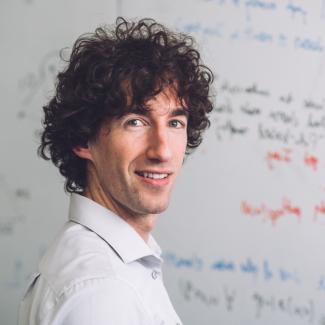Guy Bresler (Massachusetts Institute of Technology; chair)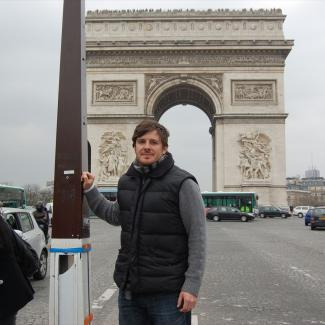Adam Klivans (University of Texas, Austin)Gábor Lugosi (Pompeu Fabra University)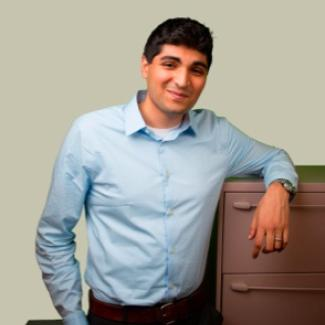Ankur Moitra (Massachusetts Institute of Technology)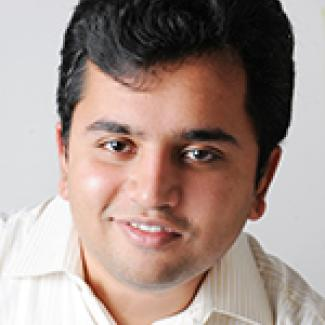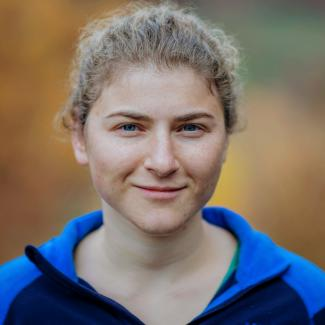Tselil Schramm (Stanford University)Yihong Wu (Yale University)

## Long-Term Participants (including Organizers)

Jean Barbier (International Center for Theoretical Physics)
Shay Moran (Technion - Israel Institute for Technology)
Nati Srebro (Toyota Technological Institute at Chicago)

## Visiting Graduate Students and Postdocs

Enric Boix (Massachusetts Institute of Technology)
Yue Li (Carnegie Mellon University)
Manuel Saenz (International Center for Theoretical Physics)